# 7.1 Graphing linear equations and inequalities in one variable

 Page 1 / 1
This module is from Elementary Algebra by Denny Burzynski and Wade Ellis, Jr. In this chapter the student is shown how graphs provide information that is not always evident from the equation alone. The chapter begins by establishing the relationship between the variables in an equation, the number of coordinate axes necessary to construct its graph, and the spatial dimension of both the coordinate system and the graph. Interpretation of graphs is also emphasized throughout the chapter, beginning with the plotting of points. The slope formula is fully developed, progressing from verbal phrases to mathematical expressions. The expressions are then formed into an equation by explicitly stating that a ratio is a comparison of two quantities of the same type (e.g., distance, weight, or money). This approach benefits students who take future courses that use graphs to display information.The student is shown how to graph lines using the intercept method, the table method, and the slope-intercept method, as well as how to distinguish, by inspection, oblique and horizontal/vertical lines. Objectives of this module: understand the concept of a graph and the relationship between axes, coordinate systems, and dimension, be able to construct one-dimensional graphs.

## Overview

• Graphs
• Axes, Coordinate Systems, and Dimension
• Graphing in One Dimension

## Graphs

We have, thus far in our study of algebra, developed and used several methods for obtaining solutions to linear equations in both one and two variables. Quite often it is helpful to obtain a picture of the solutions to an equation. These pictures are called graphs and they can reveal information that may not be evident from the equation alone.

## The graph of an equation

The geometric representation (picture) of the solutions to an equation is called the graph of the equation.

## Axis

The basic structure of the graph is the axis . It is with respect to the axis that all solutions to an equation are located. The most fundamental type of axis is the number line .

## The number line is an axis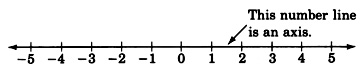We have the following general rules regarding axes.

## Number of variables and number of axes

• An equation in one variable requires one axis.
• An equation in two variables requires two axes.
• An equation in three variables requires three axes.
• ... An equation in $n$ variables requires $n$ axes.

We shall always draw an axis as a straight line, and if more than one axis is required, we shall draw them so they are all mutually perpendicular (the lines forming the axes will be at $90°$ angles to one another).

## Coordinate system

A system of axes constructed for graphing an equation is called a coordinate system .

## The phrase, graphing an equation

The phrase graphing an equation is used frequently and should be interpreted as meaning geometrically locating the solutions to an equation.

## Relating the number of variables and the number of axes

We will not start actually graphing equations until Section [link] , but in the following examples we will relate the number of variables in an equation to the number of axes in the coordinate system.

• If we wish to graph the equation $5x+2=17$ , we would need to construct a coordinate system consisting of a single axis (a single number line) since the equation consists of only one variable. We label the axis with the variable that appears in the equation.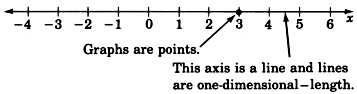We might interpret an equation in one variable as giving information in one-dimensional space. Since we live in three-dimensional space, one-dimensional space might be hard to imagine. Objects in one-dimensional space would have only length, no width or depth.

• To graph an equation in two variables such as $y=2x–3$ , we would need to construct a coordinate system consisting of two mutually perpendicular number lines (axes). We call the intersection of the two axes the origin and label it with a 0. The two axes are simply number lines; one drawn horizontally, one drawn vertically.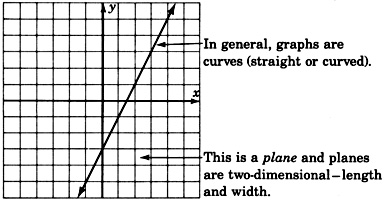Recall that an equation in two variables requires a solution to be a pair of numbers. The solutions can be written as ordered pairs $\left(x,y\right)$ . Since the equation $y=2x–3$ involves the variables $x$ and $y$ , we label one axis $x$ and the other axis $y$ . In mathematics it is customary to label the horizontal axis with the independent variable and the vertical axis with the dependent variable.

We might interpret equations in two variables as giving information in two-dimensional space. Objects in two-dimensional space would have length and width, but no depth.

• An equation in three variables, such as $3{x}^{2}–4{y}^{2}+5z=0$ , requires three mutually perpendicular axes, one for each variable. We would construct the following coordinate system and graph.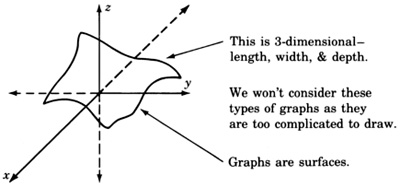We might interpret equations in three variables as giving information about three-dimensional space.

• To graph an equation in four variables, such as $3x–2y+8x–5w=–7$ , would require four mutually perpendicular number lines. These graphs are left to the imagination.

We might interpret equations in four variables as giving information in four-dimensional space. Four-dimensional objects would have length, width, depth, and some other dimension.

## Black holes

These other spaces are hard for us to imagine, but the existence of “black holes” makes the possibility of other universes of one-, two-, four-, or n -dimensions not entirely unlikely. Although it may be difficult for us “3-D” people to travel around in another dimensional space, at least we could be pretty sure that our mathematics would still work (since it is not restricted to only three dimensions)!

## Graphing in one dimension

Graphing a linear equation in one variable involves solving the equation, then locating the solution on the axis (number line), and marking a point at this location. We have observed that graphs may reveal information that may not be evident from the original equation. The graphs of linear equations in one variable do not yield much, if any, information, but they serve as a foundation to graphs of higher dimension (graphs of two variables and three variables).

## Sample set a

Graph the equation $3x–5=10$ .

Solve the equation for $x$ and construct an axis. Since there is only one variable, we need only one axis. Label the axis $x$ .

$\begin{array}{lll}3x–5\hfill & =\hfill & 10\hfill \\ 3x\hfill & =\hfill & 15\hfill \\ x\hfill & =\hfill & 5\hfill \end{array}$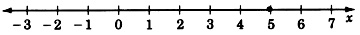Graph the equation $3x+4+7x–1+8=31$ .

Solving the equation we get,

$\begin{array}{lll}10x+11\hfill & =\hfill & 31\hfill \\ 10x\hfill & =\hfill & 20\hfill \\ x\hfill & =\hfill & 2\hfill \end{array}$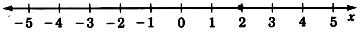## Practice set a

Graph the equation $4x+1=-7$ .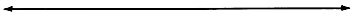$x=-2$## Sample set b

Graph the linear inequality $4x\ge 12$ .

We proceed by solving the inequality.

$\begin{array}{llll}4x\hfill & \ge \hfill & 12\hfill & \text{Divide each side by 4}\text{.}\hfill \\ x\hfill & \ge \hfill & 3\hfill & \hfill \end{array}$

As we know, any value greater than or equal to 3 will satisfy the original inequality. Hence we have infinitely many solutions and, thus, infinitely many points to mark off on our graph.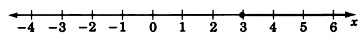The closed circle at 3 means that 3 is included as a solution. All the points beginning at 3 and in the direction of the arrow are solutions.

Graph the linear inequality $–2y–1>3$ .

We first solve the inequality.

$\begin{array}{llll}–2y–1\hfill & >\hfill & 3\hfill & \hfill \\ –2y\hfill & >\hfill & 4\hfill & \hfill \\ y\hfill & <\hfill & -2\hfill & \text{The inequality symbol reversed direction because we divided by –2}\text{.}\hfill \end{array}$

Thus, all numbers strictly less than $-2$ will satisfy the inequality and are thus solutions.

Since $-2$ itself is not to be included as a solution, we draw an open circle at $-2$ . The solutions are to the left of $-2$ so we draw an arrow pointing to the left of $-2$ to denote the region of solutions.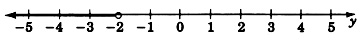Graph the inequality $–2\le y+1<1$ .

We recognize this inequality as a compound inequality and solve it by subtracting 1 from all three parts.

$\begin{array}{l}–2\le y+1<1\\ –3\le y<0\end{array}$

Thus, the solution is all numbers between $-3$ and 0, more precisely, all numbers greater than or equal to $-3$ but strictly less than 0.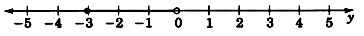Graph the linear equation $5x=–125$ .

The solution is $x=–25$ . Scaling the axis by units of 5 rather than 1, we obtain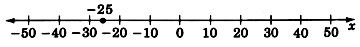## Practice set b

Graph the inequality $3x\le 18$ .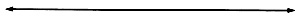$x\le 6$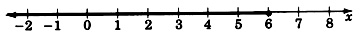Graph the inequality $-3m+1<13$ .$m>-4$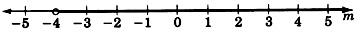Graph the inequality $-3\le x-5<5$ .$2\le x<10$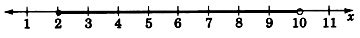Graph the linear equation $-6y=480$ .$y=-80$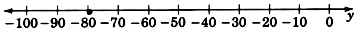## Exercises

For problems 1 - 25, graph the linear equations and inequalities.

$4x+7=19$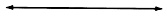$x=3$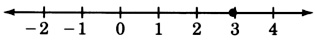$8x-1=7$$2x+3=4$$x=\frac{1}{2}$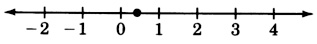$x+3=15$$6y+3=y+8$$y=1$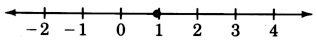$2x=0$$4+1-4=3z$$z=\frac{1}{3}$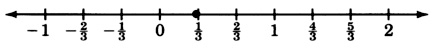$x+\frac{1}{2}=\frac{4}{3}$$7r=\frac{1}{4}$$r=\frac{1}{28}$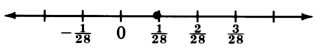$2x-6=\frac{2}{5}$$x+7\le 12$$x\le 5$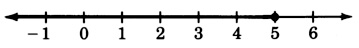$y-5<3$$x+19>2$$x>-17$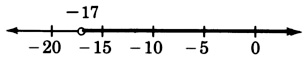$z+5>11$$3m-7\le 8$$m\le 5$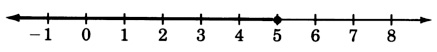$-5t\ge 10$$-8x-6\ge 34$$x\le -5$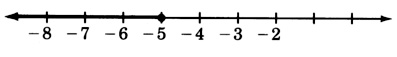$\frac{x}{4}<2$$\frac{y}{7}\le 3$$y\le 21$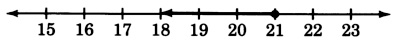$\frac{2y}{9}\ge 4$$\frac{-5y}{8}\le 4$$y\ge -\frac{32}{5}$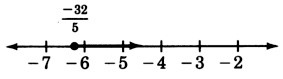$\frac{-6a}{7}<-4$$-1\le x-3<0$$2\le x<3$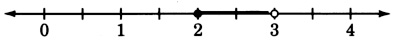$6\le x+4\le 7$$-12<-2x-2\le -8$$3\le x<5$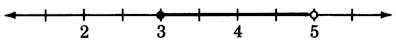## Exercises for review

( [link] ) Simplify ${\left(3{x}^{8}{y}^{2}\right)}^{3}$ .

( [link] ) List, if any should appear, the common factors in the expression $10{x}^{4}-15{x}^{2}+5{x}^{6}$ .

$5{x}^{2}$

( [link] ) Solve the inequality $-4\left(x+3\right)<-3x+1$ .

( [link] ) Solve the equation $y=-5x+8\text{\hspace{0.17em}}\text{if}\text{\hspace{0.17em}}x=-2$ .

$\left(-2,18\right)$

( [link] ) Solve the equation $2y=5\left(3x+7\right)\text{\hspace{0.17em}}\text{if}\text{\hspace{0.17em}}x=-1$ .

where we get a research paper on Nano chemistry....?
what are the products of Nano chemistry?
There are lots of products of nano chemistry... Like nano coatings.....carbon fiber.. And lots of others..
learn
Even nanotechnology is pretty much all about chemistry... Its the chemistry on quantum or atomic level
learn
da
no nanotechnology is also a part of physics and maths it requires angle formulas and some pressure regarding concepts
Bhagvanji
Preparation and Applications of Nanomaterial for Drug Delivery
revolt
da
Application of nanotechnology in medicine
what is variations in raman spectra for nanomaterials
I only see partial conversation and what's the question here!
what about nanotechnology for water purification
please someone correct me if I'm wrong but I think one can use nanoparticles, specially silver nanoparticles for water treatment.
Damian
yes that's correct
Professor
I think
Professor
Nasa has use it in the 60's, copper as water purification in the moon travel.
Alexandre
nanocopper obvius
Alexandre
what is the stm
is there industrial application of fullrenes. What is the method to prepare fullrene on large scale.?
Rafiq
industrial application...? mmm I think on the medical side as drug carrier, but you should go deeper on your research, I may be wrong
Damian
How we are making nano material?
what is a peer
What is meant by 'nano scale'?
What is STMs full form?
LITNING
scanning tunneling microscope
Sahil
how nano science is used for hydrophobicity
Santosh
Do u think that Graphene and Fullrene fiber can be used to make Air Plane body structure the lightest and strongest. Rafiq
Rafiq
what is differents between GO and RGO?
Mahi
what is simplest way to understand the applications of nano robots used to detect the cancer affected cell of human body.? How this robot is carried to required site of body cell.? what will be the carrier material and how can be detected that correct delivery of drug is done Rafiq
Rafiq
if virus is killing to make ARTIFICIAL DNA OF GRAPHENE FOR KILLED THE VIRUS .THIS IS OUR ASSUMPTION
Anam
analytical skills graphene is prepared to kill any type viruses .
Anam
Any one who tell me about Preparation and application of Nanomaterial for drug Delivery
Hafiz
what is Nano technology ?
write examples of Nano molecule?
Bob
The nanotechnology is as new science, to scale nanometric
brayan
nanotechnology is the study, desing, synthesis, manipulation and application of materials and functional systems through control of matter at nanoscale
Damian
Is there any normative that regulates the use of silver nanoparticles?
what king of growth are you checking .?
Renato
What fields keep nano created devices from performing or assimulating ? Magnetic fields ? Are do they assimilate ?
why we need to study biomolecules, molecular biology in nanotechnology?
?
Kyle
yes I'm doing my masters in nanotechnology, we are being studying all these domains as well..
why?
what school?
Kyle
biomolecules are e building blocks of every organics and inorganic materials.
Joe
Please keep in mind that it's not allowed to promote any social groups (whatsapp, facebook, etc...), exchange phone numbers, email addresses or ask for personal information on QuizOver's platform.ByByBy OpenStaxBy Szilárd JankóBy Katie MontroseBy OpenStaxBy Brooke DelaneyBy OpenStaxBy OpenStaxBy Tamsin KnoxBy Stephen VoronBy Jonathan Long